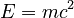### September 27th, 1905On this date in 1905, German physics journal Annalen der Physik published what would become one of the world’s most famous mathematical equations.

This issue of the journal included Albert Einstein’s paper “Does the Inertia of a Body Depend Upon Its Energy Content?”. Within this paper was the equation. While most people have heard this equation and can trace it back to Einstein, few understand what it means.

Einstein’s theory behind this equation basically means that any object that has mass has an equal amount of energy, and vice versa. To simplify this even more, energy and mass are interchangeable.

### Let’s breakdown the equation:The equation states that energy equals mass times the speed of light, squared. You might be wondering why we’re talking about the speed of light all of the sudden. When you convert a piece of matter into energy, the resulting energy is technically moving at the speed of light! According to this pbs article, “Pure energy is electromagnetic radiation—whether light or X-rays or whatever—and electromagnetic radiation travels at a constant speed of 300,000 km/sec (186,000 miles/sec)”.